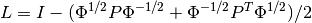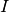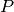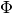Warning

This documents an unmaintained version of NetworkX. Please upgrade to a maintained version and see the current NetworkX documentation.

# directed_laplacian_matrix¶

directed_laplacian_matrix(G, nodelist=None, weight='weight', walk_type=None, alpha=0.95)[source]

Return the directed Laplacian matrix of G.

The graph directed Laplacian is the matrixwhereis the identity matrix,is the transition matrix of the graph, anda matrix with the Perron vector ofin the diagonal and zeros elsewhere.

Depending on the value of walk_type,can be the transition matrix induced by a random walk, a lazy random walk, or a random walk with teleportation (PageRank).

Parameters: G (DiGraph) – A NetworkX graph nodelist (list, optional) – The rows and columns are ordered according to the nodes in nodelist. If nodelist is None, then the ordering is produced by G.nodes(). weight (string or None, optional (default=’weight’)) – The edge data key used to compute each value in the matrix. If None, then each edge has weight 1. walk_type (string or None, optional (default=None)) – If None,is selected depending on the properties of the graph. Otherwise is one of ‘random’, ‘lazy’, or ‘pagerank’ alpha (real) – (1 - alpha) is the teleportation probability used with pagerank L – Normalized Laplacian of G. NumPy array NetworkXError – If NumPy cannot be imported NetworkXNotImplemnted – If G is not a DiGraph

Notes

Only implemented for DiGraphs

References

  Fan Chung (2005). Laplacians and the Cheeger inequality for directed graphs. Annals of Combinatorics, 9(1), 2005